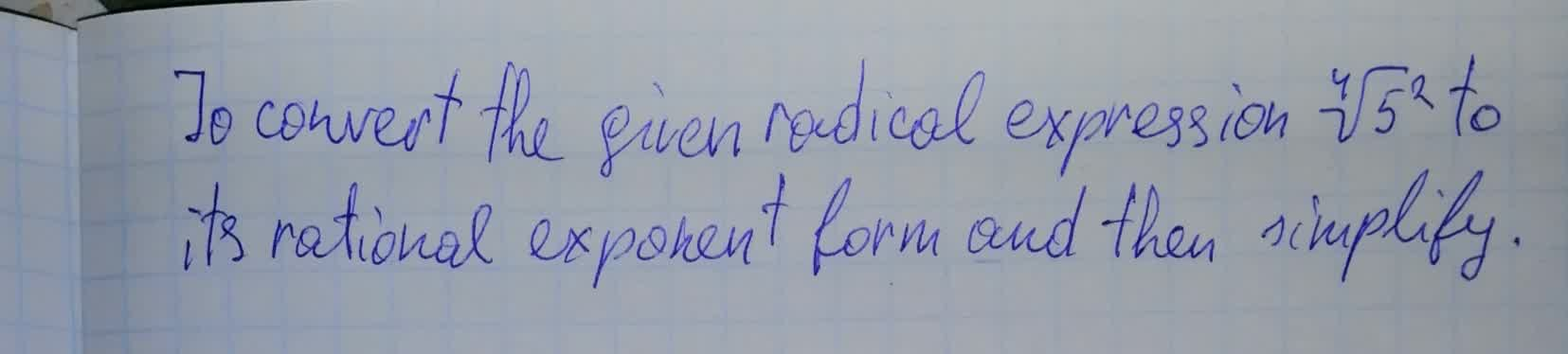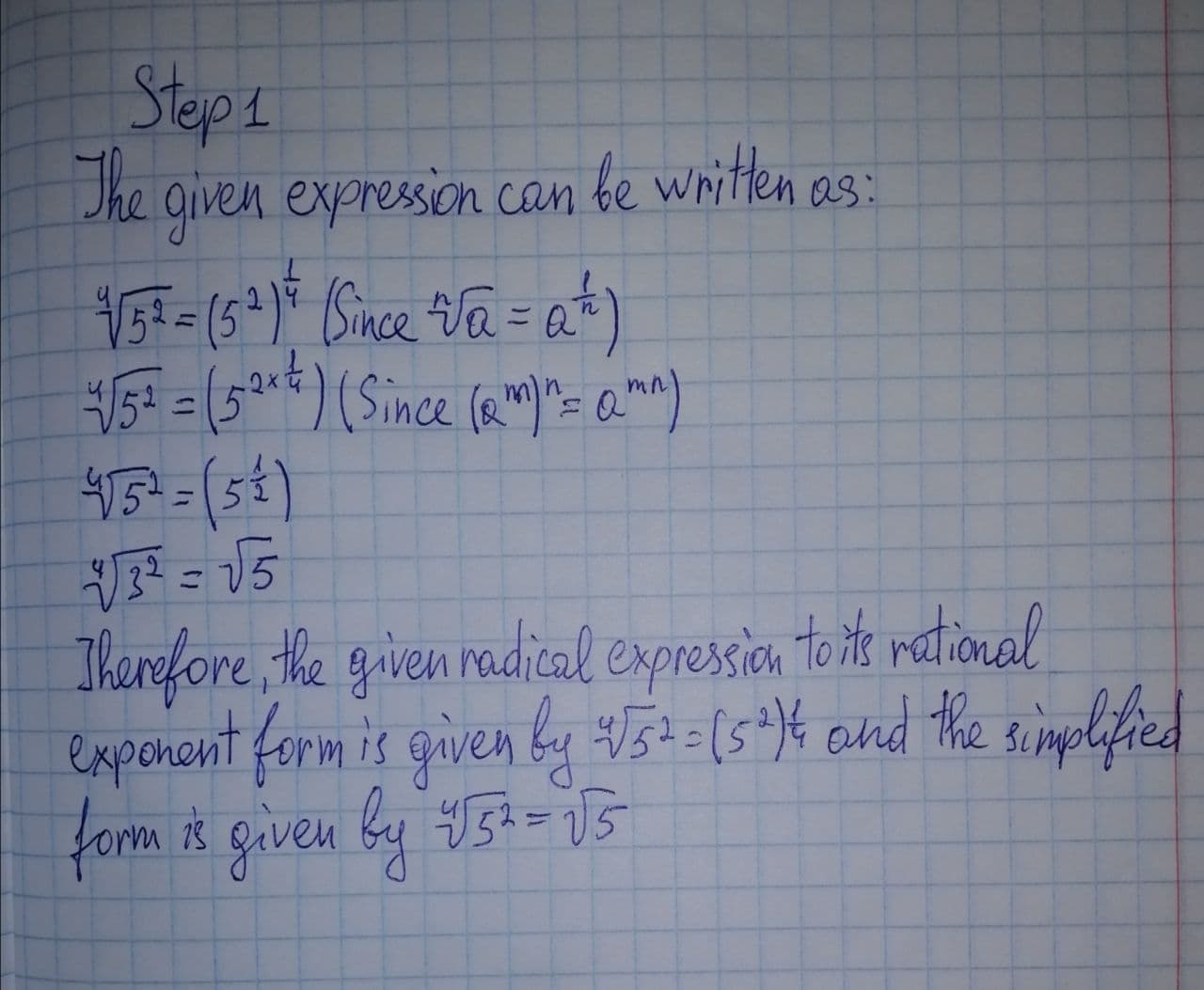Question# To convert the given radical expression \sqrt{5^2} to its rational exponent form and then simplify

Conic sections
ANSWEREDTo convert the given radical expression $$\displaystyle\sqrt{{{4}}}{\left\lbrace{5}^{{2}}\right\rbrace}$$ to its rational exponent form and then simplify